# Solving Decimal Word Problems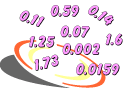Example 1: If 58 out of 100 students in a school are boys, then write a decimal for the part of the school that consists of boys.

Analysis: We can write a fraction and a decimal for the part of the school that consists of boys.

 fraction decimal0.58Example 2: A computer processes information in nanoseconds. A nanosecond is one billionth of a second. Write this number as a decimal.

Analysis: We can write a one billionth as a fraction and then as a decimal.

 fraction decimal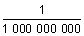0.000000001

Answer: A nanosecond, one billionth of a second, is written as 0.000000001 in decimal form.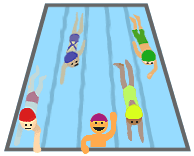Example 3: Five swimmers are entered into a competition. Four of the swimmers have had their turns. Their scores are 9.8 s, 9.75 s, 9.79 s, and 9.81 s. What score must the last swimmer get in order to win the competition?

Analysis: We must order these decimals from least to greatest. Then we must determine how the least compares with the winning score.

Step 1:

 9 . 8 0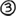9 . 7 5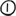9 . 7 9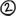9 . 8 1Step 2: The least decimal is 9.75. Now we must determine how 9.75 compares with the winning score.

Answer: The last swimmer must get a score less than 9.75 s in order to win.Example 4: To make a miniature ice cream truck, you need tires with a diameter between 1.465 cm and 1.472 cm. Will a tire that is 1.4691 cm in diameter work? Explain why or why not.

Analysis: We must compare and order these decimals to help us solve this problem. Specifically, we need to determine if the third decimal is between the first two.

Step 1: Let's start by writing one decimal beneath the other in their original order. We will place an arrow next to 1.4691 so that we can track its value.

 1 . 4 6 5 0 1 . 4 7 2 0 1 . 4 6 9 1Step 2: Now let's order these decimals from least to greatest.

 1 . 4 6 5 01 . 4 6 9 11 . 4 7 2 0Step 3: Now we must determine if the third decimal (indicated by the arrow) is between the first two.

Answer: A tire that is 1.4691 cm in diameter will work since 1.4691 is between 1.465 and 1.472.Example 5: Ellen wanted to buy the following items: A DVD player for \$49.95, a DVD holder for \$19.95 and a personal stereo for \$21.95. Does Ellen have enough money to buy all three items if she has \$90 with her?

Analysis: The phrase enough money tells us that we need to estimate the sum of the three items. We will estimate the sum by rounding each decimal to the nearest one. We must then compare our estimated sum with \$90 to see if she has enough money to buy these items.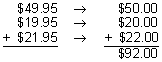Answer: No, because rounding each decimal to the nearest one, we get an estimate of \$92, and Ellen only has \$90 with her.Example 6: In Example 5, did we overestimate or underestimate? Explain your answer.

Analysis: To determine if the estimate in Example 5 is an overestimate or an underestimate, we must compare her estimate with the actual sum.

Answer: In Example 5, the estimate of \$92 is an overestimate since it is greater than \$91.85, the amount of money Ellen needs to buy these items.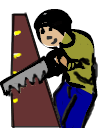Example 7: What is the combined thickness of these five shims: 0.008, 0.125, 0.15, 0.185, and 0.005 cm?

Analysis: The phrase combined thickness tells us that we need to add these five decimals to get their sum.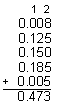Answer: The combined thickness of these five shims is 0.473 cm.Example 8: Melissa purchased \$39.46 in groceries at a store. The cashier gave her \$1.46 in change from a \$50 bill. Melissa gave the cashier an angry look. What did the cashier do wrong?

Analysis: We need to estimate the difference to see if the cashier made a mistake.

\$50.00 - \$40.00 = \$10.00

Estimate: \$1.46 is much smaller than the estimated difference of \$10.00. So the cashier must have given Melissa the wrong change.Example 9: In Example 8, how much change should Melissa get from the cashier? T

Analysis: We need to find the difference of \$50 and \$39.46.Answer: \$50.00 - \$39.46 = \$10.54Example 10: If a 10-foot piece of electrical tape has 0.037 feet cut from it, then what is the new length of the tape?.46

Analysis: We need to subtract: 10 - 0.037 and express the answer with proper units.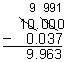Answer: 10 ft. - 0.037 ft. = 9.963 ft.

Summary: In this lesson we learned how to solve word problems involving decimals. We used the following skills to solve these problems: reading and writing decimals, comparing and ordering decimals, estimating decimal sums and differences, and adding and subtracting decimals.

### Exercises

Directions: Read each question below. You may use paper and pencil to help you solve these problems. Click once in an ANSWER BOX and type in your answer; then click ENTER. After you click ENTER, a message will appear in the RESULTS BOX to indicate whether your answer is correct or incorrect. To start over, click CLEAR.

 1.A director replayed 231 of the 1000 scenes filmed for a movie. Write a decimal for the part of the movie the director replayed. ANSWER BOX:   RESULTS BOX:
 2.The times for three runners in a 100-yard dash are 9.85 s, 9.6 s, and 9.625 s. What is the winning time? ANSWER BOX:  s  RESULTS BOX:
 3.A cylinder is normally 3.4375 cm in diameter. If it is remade to be 0.095 cm larger, what is the new size of the cylinder? ANSWER BOX:  cm  RESULTS BOX:
 4.Brandon is training for the 200-meter dash. His best running time so far was 31.25 seconds. If Brandon wants to run the dash in 27 seconds, then about how much time must he cut in order to reach his goal? ANSWER BOX:  s  RESULTS BOX:
 5.Patricia has \$425.82 in her checking account. How much does she have in her account after she makes a deposit of \$120.75 and a withdrawal of \$185.90? ANSWER BOX: \$   RESULTS BOX: